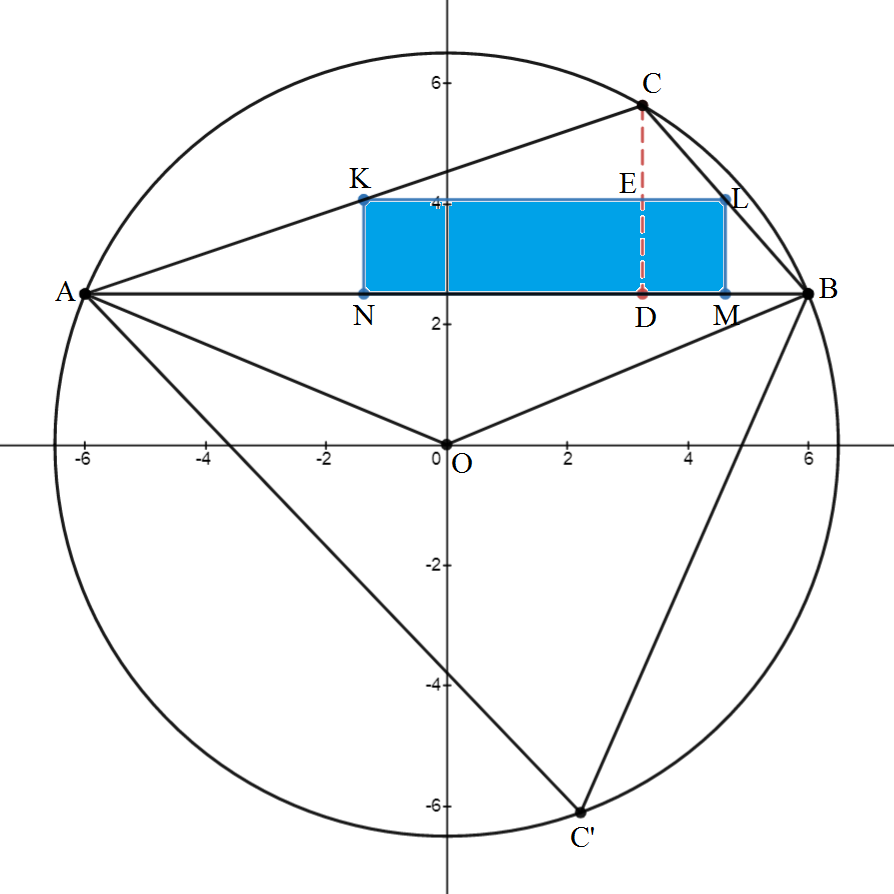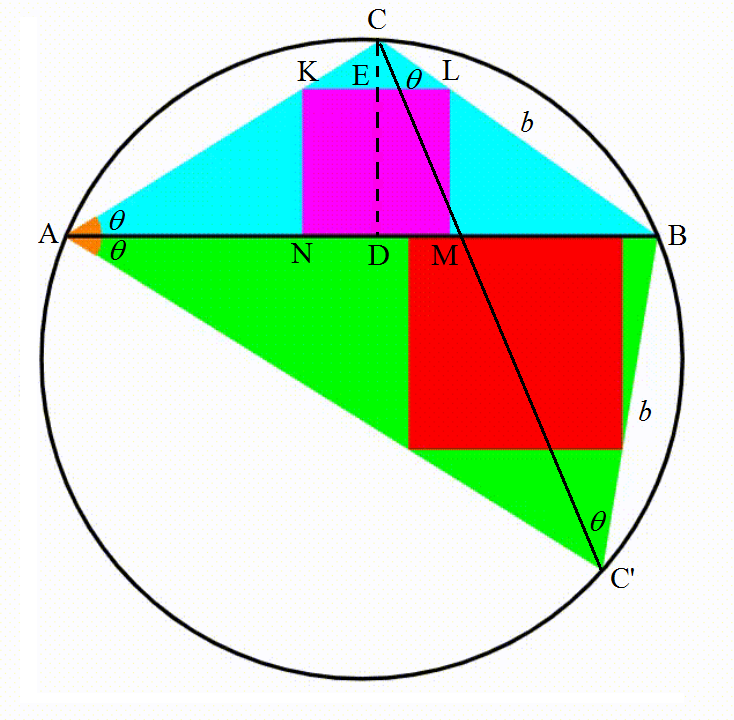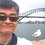# Dynamic Geometry: P96 Series

This note gives the common calculations in the solution for problems titled Dynamic Geometry: P96, 101, 106, 109, 111, 112, 113, 114, 115, 116, 117, 118, 119, 120, 125, 127, 128, and 129 so far by @Valentin Duringer. .## Radius, Chord Length, and Coordinates

Let the chord dividing a circle with center at $O$ into two circular segments of heights $4$ and $9$ be $AB$. Diameter $DE$ through $O$ cut $AB$ perpendicularly at $F$. Then $DF = 4$, $FE = 9$, and diameter $DE = DF + FE = 13$, therefore radius of the circle is $6.5$. By intersecting chords theorem $AF \cdot FB = DF \cdot FE = 4 \cdot 9 \implies AF = FB = 6 \implies AB=12$. If we set $O$ as the origin $(0,0)$ of the $xy$-plane with $AB$ parallel to the $x$-axis, then $AB$ is along $y=2.5$, and $A = (-6, 2.5)$ and $B=(6,2.5)$.

## Angles of Moving Vertices

Label the upper variable triangle as $ABC$ and the lower variable triangle $ABC'$. Since the inscribed angle $\angle AC'B$ and the central angle $\angle AOB$ both form the same arc $ACB$, $\angle AC'B = \frac 12 \angle AOB = \frac 12 \left(2 \tan^{-1} \frac {12}5 \right) = \tan^{-1} \frac {12}5$. Similarly, $\angle ACB = \pi - \tan^{-1} \frac {12}5$.

## Side Lengths of Triangle

Since the diameter of a circumcircle is given by the sine rule:

$D = \frac {AC}{\sin \angle CBA} = 13 \implies AC = 13\sin \angle CBA$

Similarly, $BC = 13 \sin \angle CAB$, $AC' = 13\sin \angle C'BA$, and $BC' = 13 \sin \angle C'AB$,

## The Largest Rectangle Inscribed by a Triangle

Consider any $\triangle ABC$ with a height $CD = h$ and a width of $AB=w$. Let the rectangle $\triangle ABC$ inscribes be $KLMN$ and the height of $\triangle CKL$ be $CE = \alpha h$, where $0 \le \alpha \le 1$. Then the area of rectangle $KLMN$, $A = KL \cdot LM$. Since $\triangle CKL$ and $\triangle ABC$ are similar $KL = \alpha \cdot AB = \alpha w$. And $LM = CD - CE = h - \alpha h$. Then $A = \alpha (1-\alpha) wh$. By AM-GM inequality, $2\sqrt{\alpha(1-\alpha)} \le \alpha + (1 - \alpha) = 1$, and equality occurs when $\alpha = 1 - \alpha \implies \alpha = \frac 12$. Then the rectangle has a maximum area $A_{\max} = \frac 14 wh$, when the height and breadth of the rectangle are half of that of the triangle.

## The Square Inscribed by a TriangleLet us consider the relationship between the side length $s$ of a square $KLMN$ to the height $CD=h$ and width $AB=w$ of the triangle $\triangle ABC$ that inscribes it. Note that $\triangle KLC$ and $\triangle ABC$ are similar. Let the height of $\triangle KLC$ be $CE$, then $CE = \dfrac swh$. From $CD = CE + ED$, we have

$h = \frac swh + s \implies s = \frac h{1+\frac hw} = \frac {wh}{h+w} = \frac {12h}{h+12} \quad \small \blue{\text{Since }w=AB=12}$

Similarly for the square in $\triangle ABC'$, $s' = \dfrac {12h'}{h'+12}$.Note by Chew-Seong Cheong
3 months, 2 weeks ago

This discussion board is a place to discuss our Daily Challenges and the math and science related to those challenges. Explanations are more than just a solution — they should explain the steps and thinking strategies that you used to obtain the solution. Comments should further the discussion of math and science.

When posting on Brilliant:

• Use the emojis to react to an explanation, whether you're congratulating a job well done , or just really confused .
• Ask specific questions about the challenge or the steps in somebody's explanation. Well-posed questions can add a lot to the discussion, but posting "I don't understand!" doesn't help anyone.
• Try to contribute something new to the discussion, whether it is an extension, generalization or other idea related to the challenge.

MarkdownAppears as
*italics* or _italics_ italics
**bold** or __bold__ bold
- bulleted- list
• bulleted
• list
1. numbered2. list
1. numbered
2. list
Note: you must add a full line of space before and after lists for them to show up correctly
paragraph 1paragraph 2

paragraph 1

paragraph 2

[example link](https://brilliant.org)example link
> This is a quote
This is a quote
    # I indented these lines
# 4 spaces, and now they show
# up as a code block.

print "hello world"
# I indented these lines
# 4 spaces, and now they show
# up as a code block.

print "hello world"
MathAppears as
Remember to wrap math in $$ ... $$ or $ ... $ to ensure proper formatting.
2 \times 3 $2 \times 3$
2^{34} $2^{34}$
a_{i-1} $a_{i-1}$
\frac{2}{3} $\frac{2}{3}$
\sqrt{2} $\sqrt{2}$
\sum_{i=1}^3 $\sum_{i=1}^3$
\sin \theta $\sin \theta$
\boxed{123} $\boxed{123}$

Sort by:

Nice work sir !

- 3 months, 2 weeks ago

Actually, the coordinate system I use is different, I use point A(0,0)

- 3 months, 2 weeks ago